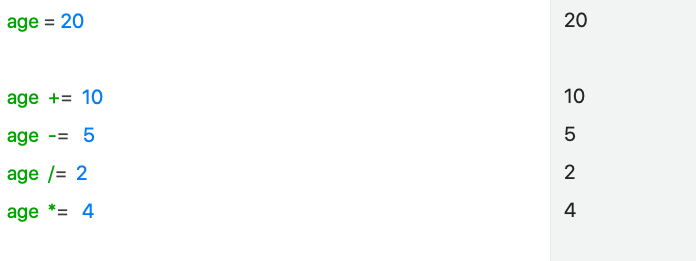Would be great to add Assignment Operators:
= x = y
+= x += y
-= x -= y
= x
= y
/= x /= y
%= x %= y
= x
= y